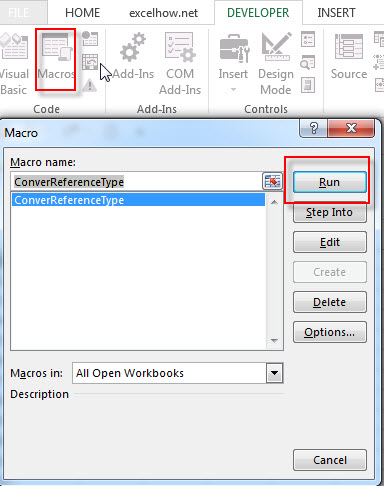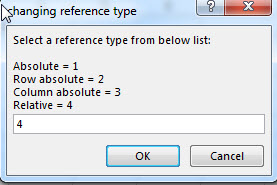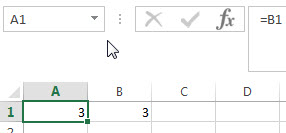# How to Convert Absolute Reference to Relative Reference

This post will guide you how to convert the cell reference from absolute reference to relative reference in excel. How to change the absolute reference of cells to relative using VBA macros in excel. How to change the reference to absolute reference to keep excel formulas while sorting cells that contain formulas.

## Convert reference from absolute to relative

The first way is that you can press F4 key to change cell reference from absolute to relative. Just refer to the following steps:

1# select the cell that contains the reference you want to change.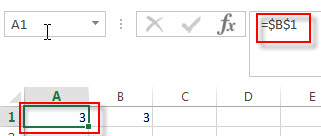2# select the reference of references that you want to convert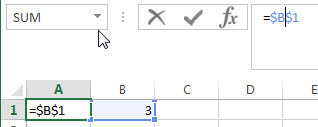3# press F4 key three times.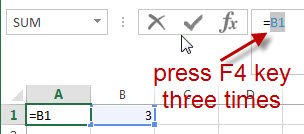Note: if you type a relative reference into formula box, then press F4, the reference will change to absolute. When you press F4 again, the reference changes to mixed type with the row fixed. So you need to press F4 key three times in the above steps.

You will see that the cell reference has been changed to relative reference.

## Convert reference from relative to absolute

The cell references are set up as relative by default in Excel. It means that when you copy the cell contains formula to another cell, the cell reference will be changed automatically. If the original cell use the absolute reference, the formula can be kept without changed.

The simplest method is that you just need to press F4 key on the selected relative reference in formula box.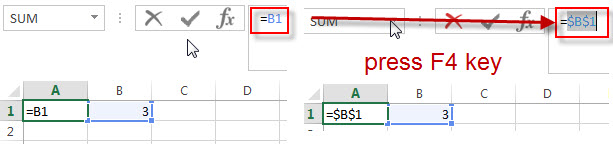## Convert reference from absolute to relative using VBA macro

You can also write an excel macro to quickly change the type of cell reference from absolute to relative. Refer to the following steps:

1# click on “Visual Basic” command under DEVELOPER Tab.2# then the “Visual Basic Editor” window will appear.

3# click “Insert” ->”Module” to create a new module4# paste the below VBA code into the code window. Then clicking “Save” button.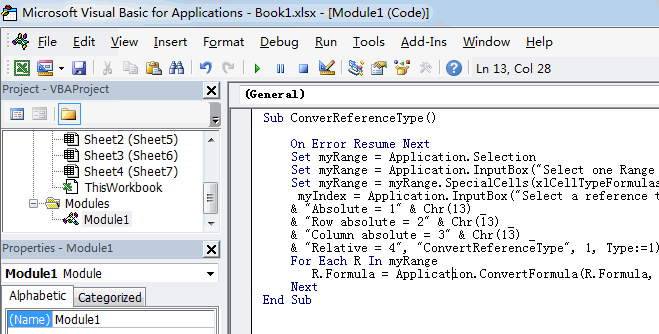```Sub ConverReferenceType()
On Error Resume Next
Set myRange = Application.Selection
Set myRange = Application.InputBox("Select one Range that you want to covert reference type:", "ConvertReferenceType", myRange.Address, Type:=8)
Set myRange = myRange.SpecialCells(xlCellTypeFormulas)
myIndex = Application.InputBox("Select a reference type from below list:" & Chr(13) & Chr(13) _
& "Absolute = 1" & Chr(13) _
& "Row absolute = 2" & Chr(13) _
& "Column absolute = 3" & Chr(13) _
& "Relative = 4", "ConvertReferenceType", 1, Type:=1)
For Each R In myRange
R.Formula = Application.ConvertFormula(R.Formula, XlReferenceStyle.xlA1, XlReferenceStyle.xlA1, myIndex)
Next
End Sub```

5# back to the current worksheet, then run the above excel macro.